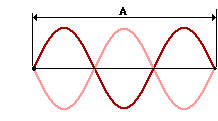Waves, Sound and Light: Wave Basics

Wave Basics: Audio Guided Solution

Problem 18:A rope is held tightly and shook until the standing wave pattern shown in the diagram at the right is established within the rope. The distance A in the diagram is 3.27 meters. The speed at which waves move along the rope is 2.62 m/s.
a. Determine the frequency of the waves creating the standing wave pattern.
b. Determine the number of vibrational cycles which would be measured in 20.0 seconds.

Audio Guided Solution

Click to show or hide the answer!

Habits of an Effective Problem Solver

• Read the problem carefully and develop a mental picture of the physical situation. If necessary, sketch a simple diagram of the physical situation to help you visualize it.
• Identify the known and unknown quantities and record in an organized manner, often times they can be recorded on the diagram itself. Equate given values to the symbols used to represent the corresponding quantity (e.g., v = 12.8 m/s, λ = 4.52 m, f = ???).
• Use physics formulas and conceptual reasoning to plot a strategy for solving for the unknown quantity.
• Identify the appropriate formula(s) to use.
• Perform substitutions and algebraic manipulations in order to solve for the unknown quantity.